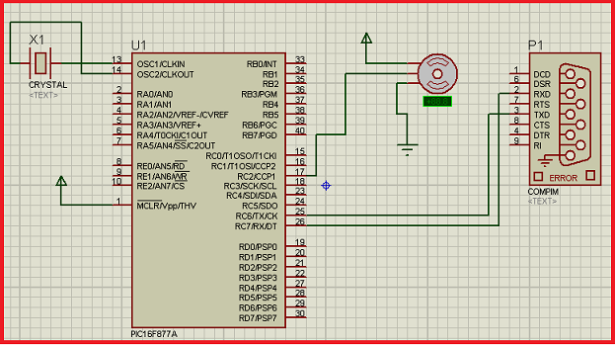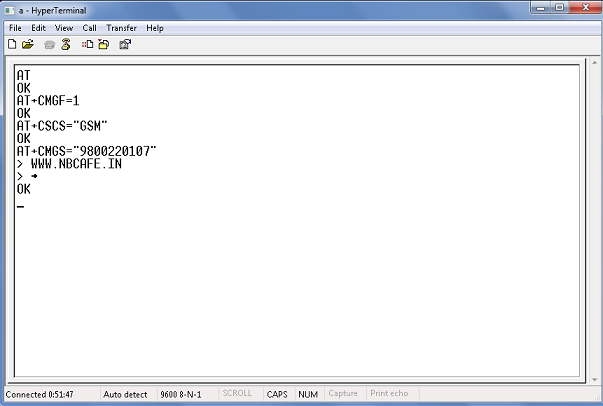# Servo motor control by Microcontroller PIC16f877 and MATLAB GUI

Here you see how a Servo motor controls by Microcontroller PIC16f877 and MATLAB GUI? It is well known to us that now a day’s use of servo motor extended in the field of Robotics. Servo motor can rotate with definite angle and we can easily use it in design of robot arm movements. And also it can use extendedly in Remote-car and Helicopters. Now in my project “Servo motor control by Microcontroller PIC16f877 and MATLAB GUI” I will show you how a servo motor is rotate with 15° interval and also control by MATLAB GUI and also show you how it simulate in Proteus with help of MATLAB GUI.

Software required for PROJECT:

1)      Mikro C Pro for PIC  ( For write C Program and generate HEX file)

2)      Proteus ISIS               (For simulate the project)

3)      MATLAB                      ( For making GUI and Controlling the motor rotation)

4)      Virtual Serial Port Emulator. ( to making virtual com port and pairing them)

Servo motor Control: We can control a rotation of Servo motor by PWM (Pulse Width Modulation) technique. Only changing the duty cycle of a pulse signal we can vary the rotation of servo motor with specific angle. Using Mikro C Pro we can easy sets PWM duty ratio for PIC Microcontroller. Parameter `duty` takes values from 0 to 255, where 0 is 0%, 127 is 50%, and 255 is 100% duty ratio. Other specific values for duty ratio can be calculated as `(Percent*255)/100`.In my project setting the specific value of Duty ratio to obtain specific angle in bellow I give you the table of specific Duty ratio Vs. Specific Angle.

 Duty ratio Angle in Degree 21 15 42 30 63 45 83 60 103 75 125 90 145 105 165 120 187 135 207 150 228 165 255 180

Circuit Diagram in Proteus: See the circuit diagram of Servo motor control by Microcontroller PIC16f877 and MATLAB GUI in bellow and see in figure that we feed input to servo motor from CCP1/Rc2 pin of Pic16f877. This CCP1 pin is used for PWM 1 so we have to take input from that pin only.

How to make MATLAB GUI: It is better to see the video of the process to How to make MATLAB GUI rather written the steps for that. See the bellow video.

C code for the project Servo motor control by Microcontroller PIC16f877 and MATLAB GUI:

For Write C code we have to know some command for initializing PWM unit and UART unit because we connect Matlab with proteus through UART port.

For more detail: Servo motor control by Microcontroller PIC16f877 and MATLAB GUI

#### Current Project / Post can also be found using:

• matlab & pic microcontroller base motor control
• control de motor con pic 16f877a matlab
• matlab gui code dc motor control